Next: Spin polarization effect (time-odd) Up: Core-polarization effects Previous: Mass polarization effect (time-even)

## Shape polarization effect (time-even)

This effect is well known both in the MF and particle-vibration-coupling approaches. Within a deformed MF theory (with the time-odd mean fields neglected), it corresponds to a simple fact that the s.p. energies (eigen-energies in a deformed potential) depend on the deformation in specific way, which is visualized by the standard Nilsson diagram . Indeed, in an axially deformed potential, a spherical multiplet of angular momentum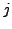splits into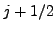orbitals according to moduli of the angular-momentum projections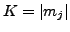. Unless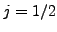, for prolate and oblate deformations, orbitals with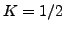decrease and increase in energy, respectively, while those with maximum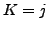behave in an opposite way. Therefore, both for prolate and oblate deformations, and for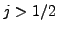, the lowest orbitals have the energies that are lower than those at the spherical point. Hence, aparticle added to a doubly-magic core always polarizes the core in such a way that the total energy decreases. On the other hand, the energy of a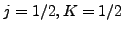orbital does not depend on deformation (in the first order), and thus such an orbital does not exert any shape polarization (in this order).

Exactly the same result is obtained in a particle-vibration-coupling model, in which aparticle can be coupled with either 0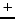or 2state of the core,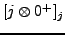or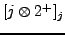, and the repulsion of these two configurations decreases the energy of the ground state with respect to the unperturbed spherical configuration. As before, for, the configurationdoes not exist, and the ground state is not lowered.

The above reasoning can be repeated for hole states, with the result that theholes added to the doubly-magic core always polarize the core in such a way that the total energy also decreases. As a consequence, the shape polarization effect decreases the s.p. energies of particle states (21) and increases those of hole states (22), and thus decreases the shell gap (24). This effect is clearly illustrated in Fig. 1.Next: Spin polarization effect (time-odd) Up: Core-polarization effects Previous: Mass polarization effect (time-even)
Jacek Dobaczewski 2008-05-18